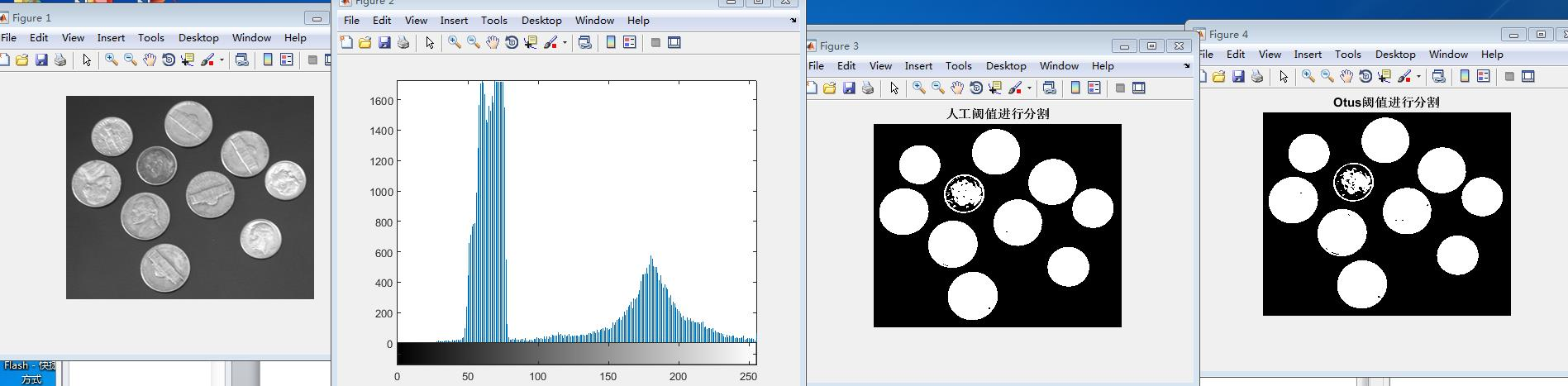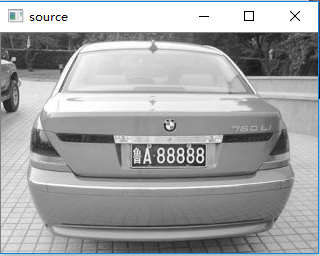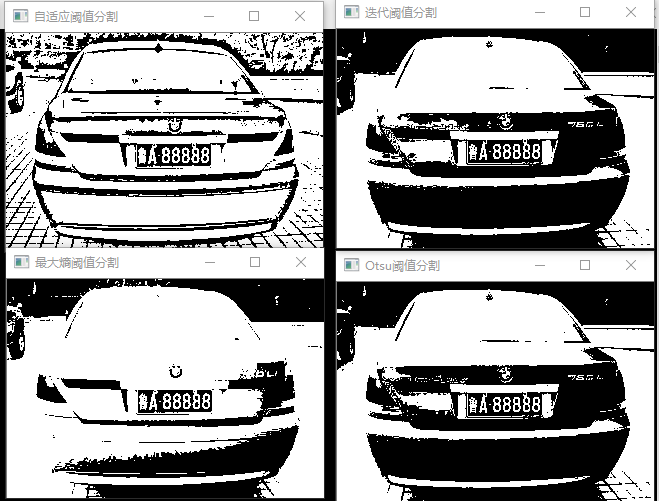# 图像处理阈值分割代码

## Matlab实现图像阈值分割

2017-11-13 15:40:22 qq_33414271 阅读数 33295
• ###### matlab 基于阈值分割的区域生长的医学图像分割3讲

matlab 基于阈值分割的区域生长的医学图像分割3讲 </p>

3课时 23分钟 614人学习 刘昱显
免费试看

1、 打开Matlab内容自带的coins.png图像。
2、 观察它的直方图。
3、 人工选定一个阈值，并进行分割。
4、 使用自动化阈值选定方法，进行分割。

``````clc,clear
imshow(I);
%输出直方图
figure;imhist(I);
%人工选定阈值进行分割，选择阈值为120
[width,height]=size(I);
T1=120;
for i=1:width
for j=1:height
if(I(i,j)<T1)
BW1(i,j)=0;
else
BW1(i,j)=1;
end
end
end
figure;imshow(BW1),title('人工阈值进行分割');
%自动选择阈值
T2=graythresh(I);
BW2=im2bw(I,T2);%Otus阈值进行分割
figure;imshow(BW2),title('Otus阈值进行分割');

``````## 图像处理基本算法 动态阈值分割

2012-02-11 16:18:32 renshengrumenglibing 阅读数 52207
• ###### matlab 基于阈值分割的区域生长的医学图像分割3讲

matlab 基于阈值分割的区域生长的医学图像分割3讲 </p>

3课时 23分钟 614人学习 刘昱显
免费试看

1．引言

2．阈值分割的基本概念

（原始图像）    （阈值分割后的二值化图像）

T(x，y，N(x，y)，f(x，y))

点相关的全局阈值T＝T(f(x，y))
(只与点的灰度值有关)

(与点的灰度值和该点的局部邻域特征有关)
局部阈值或动态阈值T＝T(x，y，N(x，y)，f(x，y))
(与点的位置、该点的灰度值和该点邻域特征有关)

1) 基于点的全局阈值方法；
2) 基于区域的全局阈值方法
3) 局部阈值方法和多阈值方法

3．基于点的全局阈值选取方法
3.1  p-分位数法
1962年Doyle提出的p-分位数法(也称p-tile法)可以说是最古老的一种阈值选取方法。该方法使目标或背景的像素比例等于其先验概率来设定阈值，简单高效，但是对于先验概率难于估计的图像却无能为力。

3.2  迭代方法选取阈值

3.3  直方图凹面分析法

Rosenfeld和Torre提出可以构造一个包含直方图 的最小凸多边形 ，由集差 确定 的凹面。若 和 分别表示 与 在灰度级之处的高度，则 取局部极大值时所对应的灰度级可以作为阈值。也有人使用低通滤波的方法平滑直方图，但是滤波尺度的选择并不容易。

3.4 最大类间方差法

，  ，  .                 (3)

在实际运用中，往往使用以下简化计算公式：
（T） ＝ WA(μa-μ)2  + Wb(μb-μ)2

3.5 熵方法

(4)

(5)

3.6 最小误差阈值

Eb(T)是目标类错分到背景类的概率，Eo(T)是背景类错分到目标类的概率

(9)

(10)

3.7 矩量保持法

3.8 模糊集方法

Pal等首先，他们把一幅具有 个灰度级的 图像看作一个模糊集 ，其中隶属函数 定义如下：
(11)

= min{μ(l),1-μ(l)}

3.9 小结

4 基于区域的全局阈值选取方法

4.1 二维熵阈值分割方法

（二维灰度直方图： 灰度－领域平均灰度）

Abutaleb，和Pal]结合Kapur]和Kirby的方法，分别提出了各自的二维熵阈值化方法，其准则函数都是使目标熵和背景熵之和最大化。Brink的方法则是使这两者中的较小者最大化，该方法的计算复杂度为 ，后来有人改进为递推快速算法将时间复杂度降为 (其中 为最大灰度级数)。

4.2  简单统计法
Kittler等人,提出一种基于简单的图像统计的阈值选取方法。使用这种方法，阈值可以直接计算得到，从而避免了分析灰度直方图，也不涉及准则函数的优化。该方法的计算公式为
(19)

4.3  直方图变化法

例如，由于目标区的象素具有一定的一致性和相关性，因此梯度值应该较小，背景区也类似。而边界区域或者噪声，就具有较大的梯度值。最简单的直方图变换方法，就是根据梯度值加权，梯度值小的象素权加大，梯度值大的象素权减小。这样，就可以使直方图的双峰更加突起，谷底更加凹陷。

4.4 其它基于区域的全局阈值法

5 局部阈值法和多阈值法

5.1 局部阈值（动态阈值）

（阈值低，对亮区效果好，则暗区差）          （阈值高，对暗区效果好，则亮区差）

（按两个区域取局部阈值的分割结果）

```/************************************************************************/
/* 全局阈值分割  自动求取阈值        */
/************************************************************************/
//自动求取阈值，增加对场景的适应性
//只需求取一次，之后就可以一直使用
#include<cv.h>
#include <highgui.h>
#include <iostream>
#include <math.h>
using namespace std;
int main(){
IplImage * image,* image2;
cvNamedWindow("image",1);
cvShowImage("image",image);
image2 = cvCreateImage(cvSize(image->width,image->height),image->depth,1);
double T = 0;
double dT0 = 1.0;//阈值求取结束标志
double dT = 255.0;

//求取平均灰度，作为阈值T的初始值T0
int i, j;
double T0 = 0,T1 = 0,T2 = 0;//初始阈值
int count1,count2;
unsigned char * ptr,*dst;
for (i = 0 ; i< image->height ; i++)
{
for (j =0 ; j < image->width;j++)
{
ptr = (unsigned char *)image->imageData + i*image->widthStep + j;
T0 += ((double)(*ptr))/image->width/image->height;
}
}
cout<<"T0:     "<<T0<<endl;
T = (int)(T0 + 0.5);
//计算T两侧的灰度平均值，然后把二者的均值赋值给T
while (dT > dT0)
{

T1 = 0;
T2 = 0;
count1 = 0;
count2 = 0;
for (i = 0 ; i< image->height ; i++)
{
for (j =0 ; j < image->width;j++)
{
ptr = (unsigned char *)image->imageData + i*image->widthStep + j;
if (*ptr > T)
{
T1 += ((double)(*ptr))/image->width/image->height;
count1++;
}
else if(*ptr < T)
{
T2 +=  ((double)(*ptr))/image->width/image->height;
count2++;
}
}
}

T1 = T1*image->width*image->height/count1;
T2 = T2*image->width*image->height/count2;
dT = fabs(T - (T1 + T2)/2);

cout<<"T1"<<T1<<endl;
cout<<"T2"<<T2<<endl;
cout<<"dT  " << dT<<endl;
T = (T1 + T2)/2;
cout<<"T:     "<<T<<endl;
}

//根据求取的阈值进行分割
for (i = 0 ; i< image2->height ; i++)
{
for (j =0 ; j < image2->width;j++)
{
ptr = (unsigned char *)image->imageData + i*image->widthStep + j;
dst = (unsigned char *)image2->imageData+i*image2->widthStep+j;
if (*ptr > T)
{
*dst = 255;
}
else
{
*dst =0;
}
}
}

cvNamedWindow("image2",1);
cvShowImage("image2",image2);
cvSaveImage("E:\\image\\dowels2.tif",image2);
cvWaitKey(0);
return 0;
}```5.1.1 阈值插值法
首先将图像分解成系列子图，由于子图相对原图很小，因此受阴影或对比度空间变化等带来的问题的影响会比较小。然后对每个子图计算一个局部阈值（此时的阈值可用任何一种固定阈值选取方法）。通过对这些子图所得到的阈值进行插值，就可以得到对原图中每个象素进行分割所需要的合理阈值。这里对应每个象素的阈值合起来构成的一个曲面，叫做阈值曲面。

5.1.2 水线阈值算法

5.1.3 其它的局部阈值法

文献 中提出一种基于局部梯度最大值的插值方法。首先平滑图像，并求得具有局部梯度最大值的像素点，然后利用这些像素点的位置和灰度在图像上内插，得到灰度级阈值表面。

5.2 多阈值法

5.2.1 基于小波的多域值方法。

5.2.2 基于边界点的递归多域值方法。

5.2.3 均衡对比度递归多域值方法。

6 阈值化算法评价简介

然而，如同所有的图像分割方法一样，阈值化结果的评价是一个比较困难的问题。事实上对图像分割本身还缺乏比较系统的精确的研究，因此对其评价则更差一些。人们先后已经提出了几十个评价准则。这些准则中又有定性的，也有定量的；有分析算法的，也有检测实验结果的，文献将它们大致分为13类。

## 图像处理——常用阈值分割方法及源码

2018-07-18 20:37:18 fly108108 阅读数 32097
• ###### matlab 基于阈值分割的区域生长的医学图像分割3讲

matlab 基于阈值分割的区域生长的医学图像分割3讲 </p>

3课时 23分钟 614人学习 刘昱显
免费试看

1、Otsu阈值分割

2、自适应阈值分割

3、 最大熵阈值分割法

4、 迭代阈值分割

5、测验

## 1、Otsu阈值分割

Otsu（大津法或最大类间方差法）使用的是聚类的思想，把图像的灰度数按灰度级分成2个部分，使得两个部分之间的灰度值差异最大，每个部分之间的灰度差异最小，通过方差的计算来寻找一个合适的灰度级别来划分。 所以可以在二值化的时候采用otsu算法来自动选取阈值进行二值化。otsu算法被认为是图像分割中阈值选取的最佳算法，计算简单，不受图像亮度和对比度的影响。因此，使类间方差最大的分割意味着错分概率最小。

opencv调用格式为：threshold(sourceImage, dstImage, 0, 255, CV_THRESH_OTSU);

sourceImage：输入图像；

dstImage：输出图像；

otsu算法的源码如下：

``````Mat OtsuAlgThreshold(Mat &image)
{
if (image.channels() != 1)
{
cout << "Please input Gray-image!" << endl;
}
int T = 0; //Otsu算法阈值
double varValue = 0; //类间方差中间值保存
double w0 = 0; //前景像素点数所占比例
double w1 = 0; //背景像素点数所占比例
double u0 = 0; //前景平均灰度
double u1 = 0; //背景平均灰度
double Histogram = { 0 }; //灰度直方图，下标是灰度值，保存内容是灰度值对应的像素点总数
uchar *data = image.data;

double totalNum = image.rows*image.cols; //像素总数

for (int i = 0; i < image.rows; i++)
{
for (int j = 0; j < image.cols; j++)
{
if (image.at<uchar>(i, j) != 0) Histogram[data[i*image.step + j]]++;
}
}
int minpos, maxpos;
for (int i = 0; i < 255; i++)
{
if (Histogram[i] != 0)
{
minpos = i;
break;
}
}
for (int i = 255; i > 0; i--)
{
if (Histogram[i] != 0)
{
maxpos = i;
break;
}
}

for (int i = minpos; i <= maxpos; i++)
{
//每次遍历之前初始化各变量
w1 = 0;       u1 = 0;       w0 = 0;       u0 = 0;
//***********背景各分量值计算**************************
for (int j = 0; j <= i; j++) //背景部分各值计算
{
w1 += Histogram[j];   //背景部分像素点总数
u1 += j*Histogram[j]; //背景部分像素总灰度和
}
if (w1 == 0) //背景部分像素点数为0时退出
{
break;
}
u1 = u1 / w1; //背景像素平均灰度
w1 = w1 / totalNum; // 背景部分像素点数所占比例
//***********背景各分量值计算**************************

//***********前景各分量值计算**************************
for (int k = i + 1; k < 255; k++)
{
w0 += Histogram[k];  //前景部分像素点总数
u0 += k*Histogram[k]; //前景部分像素总灰度和
}
if (w0 == 0) //前景部分像素点数为0时退出
{
break;
}
u0 = u0 / w0; //前景像素平均灰度
w0 = w0 / totalNum; // 前景部分像素点数所占比例
//***********前景各分量值计算**************************

//***********类间方差计算******************************
double varValueI = w0*w1*(u1 - u0)*(u1 - u0); //当前类间方差计算
if (varValue < varValueI)
{
varValue = varValueI;
T = i;
}
}
Mat dst;
threshold(image, dst, T, 255, CV_THRESH_OTSU);
return dst;
}``````

## 2、自适应阈值分割

二值化算法是用输入像素的值I与一个值C来比较，根据比较结果确定输出值。

自适应二值化的每一个像素的比较值C都不同，比较值C由这个像素为中心的一个块范围计算在减去差值delta得到。

其中，C的常用计算方法有两种：

a、平均值减去差值delta（使用盒过滤boxfilter，性能会非常不错）

b、高斯分布加权和减去差值delta (使用高斯滤波GaussionBlur)

``````void myadaptive(InputArray _src, OutputArray _dst, double maxValue,
int method, int type, int blockSize, double delta)
{
Mat src = _src.getMat();

CV_Assert(src.type() == CV_8UC1);
CV_Assert(blockSize % 2 == 1 && blockSize > 1);
Size size = src.size();

_dst.create(size, src.type());
Mat dst = _dst.getMat();

if (maxValue < 0)
{
dst = Scalar(0);
return;
}

Mat mean;
if (src.data != dst.data)
mean = dst;
{
GaussianBlur(src, mean, Size(blockSize, blockSize), 0, 0, BORDER_REPLICATE);
}
{
boxFilter(src, mean, src.type(), Size(blockSize, blockSize),
Point(-1, -1), true, BORDER_REPLICATE);
}
else
{
}

int i, j;
uchar imaxval = saturate_cast<uchar>(maxValue);
int idelta = type == THRESH_BINARY ? cvCeil(delta) : cvFloor(delta);
uchar tab;

if (type == CV_THRESH_BINARY)
for (i = 0; i < 768; i++)
tab[i] = (uchar)(i - 255 > -idelta ? imaxval : 0);
else if (type == CV_THRESH_BINARY_INV)
for (i = 0; i < 768; i++)
tab[i] = (uchar)(i - 255 <= -idelta ? imaxval : 0);
else
{
}

if (src.isContinuous() && mean.isContinuous() && dst.isContinuous())
{
size.width *= size.height;
size.height = 1;
}

for (i = 0; i < size.height; i++)
{
const uchar* sdata = src.data + src.step*i;
const uchar* mdata = mean.data + mean.step*i;
uchar* ddata = dst.data + dst.step*i;

for (j = 0; j < size.width; j++)
// 将[-255, 255] 映射到[0, 510]然后查表
ddata[j] = tab[sdata[j] - mdata[j] + 255];
}
}``````

## 3、 最大熵阈值分割法

https://blog.csdn.net/robin__chou/article/details/53931442

https://blog.csdn.net/xw20084898/article/details/22760169

``````Mat EntropySeg(Mat src)
{
int tbHist = { 0 };
int index = 0;
double Property = 0.0;
double maxEntropy = -1.0;
double frontEntropy = 0.0;
double backEntropy = 0.0;
int TotalPixel = 0;
int nCol = src.cols*src.channels();
for (int i = 0; i < src.rows; i++)
{
uchar* pData = src.ptr<uchar>(i);
for (int j = 0; j < nCol; j++)
{
++TotalPixel;
tbHist[pData[j]] += 1;
}
}

for (int i = 0; i < 256; i++)
{
double backTotal = 0;
for (int j = 0; j < i; j++)
{
backTotal += tbHist[j];
}

for (int j = 0; j < i; j++)
{
if (tbHist[j] != 0)
{
Property = tbHist[j] / backTotal;
backEntropy += -Property*logf((float)Property);
}
}

for (int k = i; k < 256; k++)
{
if (tbHist[k] != 0)
{
Property = tbHist[k] / (TotalPixel - backTotal);
frontEntropy += -Property * logf((float)Property);
}
}

if (frontEntropy + backEntropy > maxEntropy)
{
maxEntropy = frontEntropy + backEntropy;
index = i;
}

frontEntropy = 0.0;
backEntropy = 0.0;
}

Mat dst;
threshold(src, dst, index, 255, 0);
return dst;
}``````

## 4、 迭代阈值分割

（1）选择灰度图的平均值作为初始阈值T0 ;

（2）计算小于等于T0的平均值T1, 和大于T0的平均值T2;

（3）新的阈值为T = （T1 + T2）/ 2;

（4）比较T和T0,若相等，则返回T，即为迭代阈值; 否则 T0 = T,重复（1）-（3）

``````Mat IterationThreshold(Mat src)
{
int width = src.cols;
int height = src.rows;
int hisData = { 0 };
for (int j = 0; j < height; j++)
{
uchar* data = src.ptr<uchar>(j);
for (int i = 0; i < width; i++)
hisData[data[i]]++;
}

int T0 = 0;
for (int i = 0; i < 256; i++)
{
T0 += i*hisData[i];
}
T0 /= width*height;

int T1 = 0, T2 = 0;
int num1 = 0, num2 = 0;
int T = 0;
while (1)
{
for (int i = 0; i < T0 + 1; i++)
{
T1 += i*hisData[i];
num1 += hisData[i];
}
if (num1 == 0)
continue;
for (int i = T0 + 1; i < 256; i++)
{
T2 += i*hisData[i];
num2 += hisData[i];
}
if (num2 == 0)
continue;

T = (T1 / num1 + T2 / num2) / 2;

if (T == T0)
break;
else
T0 = T;
}

Mat dst;
threshold(src, dst, T, 255, 0);
return dst;
}``````

## 5、测验

``````void main()
{
cvtColor(src, src, COLOR_RGB2GRAY);

Mat bw1, bw2, bw3, bw4;
bw2 = EntropySeg(src);
bw3 = OtsuAlgThreshold(src);
bw4 = IterationThreshold(src);

imshow("source", src);
imshow("自适应阈值分割", bw1);
imshow("最大熵阈值分割", bw2);
imshow("Otsu阈值分割", bw3);
imshow("迭代阈值分割", bw4);
waitKey(0);
}``````## python数字图像处理（11）：图像自动阈值分割

2018-04-24 00:36:24 forever0_0love 阅读数 1754
• ###### matlab 基于阈值分割的区域生长的医学图像分割3讲

matlab 基于阈值分割的区域生长的医学图像分割3讲 </p>

3课时 23分钟 614人学习 刘昱显
免费试看

1、threshold_otsu

skimage.filters.threshold_otsu(imagenbins=256)

```from skimage import data,filters
import matplotlib.pyplot as plt
image = data.camera()
thresh = filters.threshold_otsu(image)   #返回一个阈值
dst =(image <= thresh)*1.0   #根据阈值进行分割

plt.figure('thresh',figsize=(8,8))

plt.subplot(121)
plt.title('original image')
plt.imshow(image,plt.cm.gray)

plt.subplot(122)
plt.title('binary image')
plt.imshow(dst,plt.cm.gray)

plt.show()```2、threshold_yen

`thresh = filters.threshold_yen(image) `3、threshold_li

`thresh = filters.threshold_li(image)`4、threshold_isodata

threshold = (image[image <= threshold].mean() +image[image > threshold].mean()) / 2.0

`thresh = filters.threshold_isodata(image)`

block_size: 块大小，指当前像素的相邻区域大小，一般是奇数（如3，5，7。。。）

method: 用来确定自适应阈值的方法，有’mean’, ‘generic’, ‘gaussian’ 和 ‘median’。省略时默认为gaussian

```from skimage import data,filters
import matplotlib.pyplot as plt
image = data.camera()

plt.figure('thresh',figsize=(8,8))

plt.subplot(121)
plt.title('original image')
plt.imshow(image,plt.cm.gray)

plt.subplot(122)
plt.title('binary image')
plt.imshow(dst,plt.cm.gray)

plt.show()``````dst1 =filters.threshold_adaptive(image,31,'mean')## [matlab图像处理] 阈值分割

2014-03-11 15:50:40 u014037422 阅读数 17523
• ###### matlab 基于阈值分割的区域生长的医学图像分割3讲

matlab 基于阈值分割的区域生长的医学图像分割3讲 </p>

3课时 23分钟 614人学习 刘昱显
免费试看

%迭代式阈值分割  otsu阈值分割  二值化
close all;%关闭所有窗口
clear;%清除变量的状态数据
clc;%清除命令行

subplot(2,2,1);
imshow(I);
title('1 rice的原图');

%迭代式阈值分割
zmax=max(max(I));%取出最大灰度值
zmin=min(min(I));%取出最小灰度值
tk=(zmax+zmin)/2;
bcal=1;
[m,n]=size(I);
while(bcal)
%定义前景和背景数
iforeground=0;
ibackground=0;
%定义前景和背景灰度总和
foregroundsum=0;
backgroundsum=0;
for i=1:m
for j=1:n
tmp=I(i,j);
if(tmp>=tk)
%前景灰度值
iforeground=iforeground+1;
foregroundsum=foregroundsum+double(tmp);
else
ibackground=ibackground+1;
backgroundsum=backgroundsum+double(tmp);
end
end
end
%计算前景和背景的平均值
z1=foregroundsum/iforeground;
z2=foregroundsum/ibackground;
tktmp=uint8((z1+z2)/2);
if(tktmp==tk)
bcal=0;
else
tk=tktmp;
end
%当阈值不再变化时,说明迭代结束
end
disp(strcat('迭代的阈值:',num2str(tk)));%在command window里显示出 :迭代的阈值:阈值
newI=im2bw(I,double(tk)/255);%函数im2bw使用阈值（threshold）变换法把灰度图像（grayscale image）
%转换成二值图像。所谓二值图像， 一般意义上是指只有纯黑（0）、纯白（255）两种颜色的图像。
%语法
%BW = im2bw(I, level)
%BW = im2bw(X, map, level)
%BW = im2bw(RGB, level)
%其中level就是设置阈值的。level取值范围[0, 1]。
subplot(2,2,2);
imshow(newI);
title('2 rice的迭代法分割效果图');

%otsu阈值分割
bw=graythresh(I);
disp(strcat('otsu阈值分割的阈值:',num2str(bw*255)));%在command window里显示出 :迭代的阈值:阈值
newII=im2bw(I,bw);
subplot(2,2,3);
imshow(newII);
title('3 rice的otsu阈值分割');

%二值化 阈值为135
[width,height,bmsize]=size(I);
for i=1:width
for j=1:height
if I(i,j)>135
I(i,j)=255;
else
I(i,j)=0;
end
end
end
subplot(2,2,4);
imshow(I);
title('4 rice的二值阈值分割');